Example Questions

Example Question #11 : Geometry

Quantitative Comparison

A circle has a radius of 2.

Quantity A: The area of the circle

Quantity B: The circumference of the circle

The two quantities are equal.

Quantity B is greater.

Quantity A is greater.

The relationship cannot be determined from the information given.

The two quantities are equal.

Explanation:

This is one of the only special cases where the area equals the circumference of the circle. The Area = πr2 = 4π.  The circumference = 2πr = 4π.

Note: For a quantitative comparison such as this one where the columns have numeric values instead of variables, the answer will rarely be "cannot be determined".

Quantitative Comparison

Quantity A: Area of a right triangle with sides 7, 24, 25

Quantity B: Area of a circle with radius 5

Quantity A is greater.

Quantity B is greater.

The relationship cannot be determined from the information given.

The two quantities are equal.

Quantity A is greater.

Explanation:

Quantity A: area = base * height / 2 = 7 * 24/2 = 84

Quantity B: area = πr2 = 25π

Now we have to remember what π is.  Using π = 3, the area is approximately 75.  Using π = 3.14, the area increases a little bit, but no matter how exact an approximation for π, this area will never be larger than Quantity A.

Example Question #1 : How To Find The Area Of A Circle

If a circular garden with a radius of 3 ft. is bordered by a circular sidewalk that is 2 ft. wide, what is the area of the sidewalk?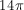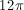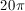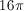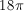Explanation:

To solve this problem, you must find the area of the entire circle (garden and sidewalk) and subtract it by the area of the inner garden. The entire area has a radius of 5 ft. (3 ft. radius of the garden plus the 2 ft. wide sidewalk), giving it an area of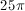. The inner garden has a radius of 3 ft. and an area of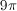. The difference is, which is the area of the sidewalk.

Example Question #1 : How To Find The Area Of A Circle

If a circular monument with a radius of 30 feet is surrounded by a circular garden that is 20 feet wide, what is the area of the garden?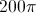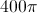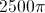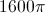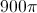Explanation:

To find the area of the garden, you need to find the entire area and subtract that by the area of the inner circle, or the monument. The radius of the larger circle is 50, which makes its area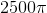. The radius of the inner circle is 30, which makes its area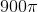. The difference is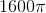.

A small circle with radius 5 lies inside a larger circle with radius x. What is the area of the region inside the larger circle, but outside of the smaller circle, in terms of x?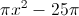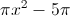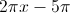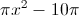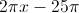Explanation:

Since the answers are in terms of pi, simply find the area of each circle in terms of x and ∏:

Smaller: ∏(5)2 = 25∏

Larger: ∏x2

We must subtract the inner circle from the outer circle; this translates to ∏x2-25∏.

Example Question #11 : Geometry

Given circle O with a diameter of 2 and square ABCD inscribed within circle O, what is the area of the shaded region?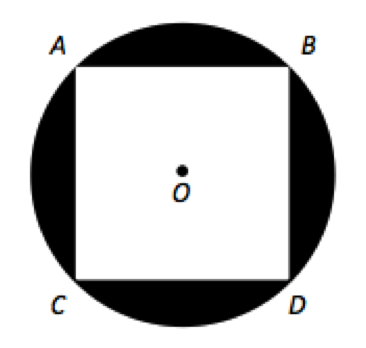2

π – 2

4

4π – 2

π – 2

Explanation:

There are two steps to this problem: determining the area of the circle and determining the area of the square. The area of the circle is πr2 which is π(2/1)2 or π. AD is a diameter of circle O and creates two isosceles right triangles with ACD and ABD. The relationship between sides of an isosceles right triangle is 1 : 1 : √2. Thus the sides of square ABCD are √2 and the area is 2. The area of the shaded region is the area of the circle minus the area of the square, or π – 2.

Example Question #3 : How To Find The Area Of A Circle

For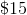, Chelsea can get either a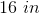diameter pizza or two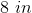diameter pizzas. Which is the better deal?twoThe two values are equal.

Cannot be determined.Explanation: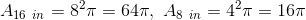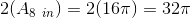Therefore the 16 inch pizza is the better deal.

Example Question #18 : Geometry

Circle B has a circumference of 36π. What is the area of circle A, which has a radius half the length of the radius of circle B?

18π

81π

324π

18

81π

Explanation:

To find the radius of circle B, use the circumference formula (c = πd = 2πr):

2πr = 36π

Divide each side by 2π: r = 18

Now, if circle A has a radius half the length of that of B, A's radius is 18 / 2 = 9.

The area of a circle is πr2.  Therefore, for A, it is π*92 = 81π.

Example Question #19 : Geometry

"O" is the center of the circle as shown below.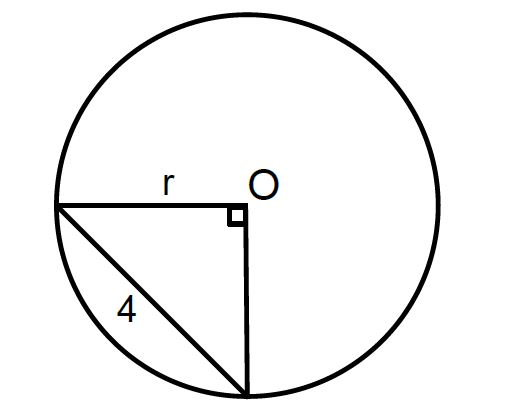A

---

B

---

3

Quanitity A is greater

The relationship cannot be determined

Quantity B is greater

The two quantities are equal

Quantity B is greater

Explanation:

We know the triangle inscribed within the circle must be isosceles, as it contains a 90-degree angle and fixed radii. As such, the opposite angles must be equal. Therefore we can use a simplified version of the Pythagorean Theorem,

a2 + a= c2 → 2r= 16 → r2 = 8; r = √8 < 3. (since we know √9 = 3, we know √8 must be less); therefore, Quantity B is greater.

Example Question #20 : Geometry

Which point could lie on the circle with radius 5 and center (1,2)?

(4,6)

(3,–2)

(–3, 6)

(4,–1)

(3,4)